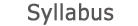2015 - 2016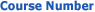0509-2805-01
Introduction to Probability and Statistics for Mechanicl & M
FACULTY OF ENGINEERING | DEPARTMENTS OF ENGINEERING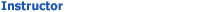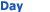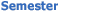Ayala Mashiah Wolfson - Engineering 238 Sun 1300-1600 Sem  1
University credit hours:  4.0

Course description
(for mechanical eng. Students)
Credit points: 3.5
Prerequisites: Differential and Integral Calculus; Linear Algebra
Introduction: Probability and statistics as models for the description of random phenomena. Basic concepts in Probability: Sample space, events, probability function, combinatorial analysis, conditional probability, total probabilities formulae, Bayes theorem, independence. Random Variables: Discrete random variable and probability function, continuous random variable and density function, cumulative distribution function, expectation and variance. Useful distributions: Binomial. Poison, Geometric, Hypergeometric, Normal, Exponential. Functions of random variables, moment generating function. Multivariate distributions: joint and marginal distributions, conditional distribution and conditional expectation, covariance and correlation, independence, distribution of sum and other functions of random variables. Limit theorems: Chebyshev inequality, laws of large numbers, central limit theorem, normal approximation to binomial. Statistical Inference: Estimation: Basic concepts in estimation, unbiased estimation, estimation methods: moments and maximum likelihood, confidence intervals for the mean. Tests of hypothesis: Basic concepts: Errors in inference, significance level, power, tests concerning the mean.

accessibility declaration